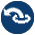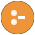#yall.pl -- Lambda expressions

Prolog realizes high-order programming with meta-calling. The core predicate of this is call/1, which simply calls its argument. This can be used to define higher-order predicates such as ignore/1 or forall/2. The call/N construct calls a closure with N-1 additional arguments. This is used to define higher-order predicates such as the maplist/N family or foldl/N.

The problem with higher order predicates based on call/N is that the additional arguments are always added to the end of the closure's argument list. This often requires defining trivial helper predicates to get the argument order right. For example, if you want to add a common postfix to a list of atoms you need to apply `atom_concat(In,Postfix,Out)`, but `maplist(x(PostFix),ListIn,ListOut)` calls `x(PostFix,In,Out)`. This is where this library comes in, which allows us to write

```?- maplist([In,Out]>>atom_concat(In,'_p',Out), [a,b], ListOut).
ListOut = [a_p, b_p].```

The `{...}` specifies which variables are shared between the lambda and the context. This allows us to write the code below. Without the `{PostFix}` a free variable would be passed to atom_concat/3.

```add_postfix(PostFix, ListIn, ListOut) :-
maplist({PostFix}/[In,Out]>>atom_concat(In,PostFix,Out),
ListIn, ListOut).```

This introduces the second application area of lambda expressions: the ability to stop binding variables in the context. This features shines when combined with bagof/3 or setof/3 where you normally have to specify the the variables in whose binding you are not interested using the `Var^Goal` construct (marking Var as existential quantified). Lambdas allow doing the reverse: specify the variables in which you are interested.

Lambda expressions use the syntax below

`{...}/[...]>>Goal.`

The `{...}` optional part is used for lambda-free variables. The order of variables doesn't matter hence the `{...}` set notation.

The `[...]` optional part lists lambda parameters. Here order of variables matters hence the list notation.

As `/` and `>>` are standard infix operators, no new operators are added by this library. An advantage of this syntax is that we can simply unify a lambda expression with Free/Parameters>>Lambda to access each of its components. Spaces in the lambda expression are not a problem although the goal may need to be written between ()'s. Goals that are qualified by a module prefix also need to be wrapped inside parentheses.

Combined with library(apply_macros), library(yall) allows writing one-liners for many list operations that have the same performance as hand written code.

The module name, yall, stands for Yet Another Lambda Library.

This module implements Logtalk's lambda expressions syntax. The development of this module was sponsored by Kyndi, Inc.

author
- Paulo Moura and Jan Wielemaker
To be done
- Extend optimization support
+Parameters >> +Lambda
>>(+Parameters, +Lambda, ?A1)
>>(+Parameters, +Lambda, ?A1, ?A2)
>>(+Parameters, +Lambda, ?A1, ?A2, ?A3)
>>(+Parameters, +Lambda, ?A1, ?A2, ?A3, ?A4)
>>(+Parameters, +Lambda, ?A1, ?A2, ?A3, ?A4, ?A5)
>>(+Parameters, +Lambda, ?A1, ?A2, ?A3, ?A4, ?A5, ?A6)
>>(+Parameters, +Lambda, ?A1, ?A2, ?A3, ?A4, ?A5, ?A6, ?A7)
Calls a copy of Lambda. This is similar to `call(Lambda,A1,...)`, but arguments are reordered according to the list Parameters:
• The first `length(Parameters)` arguments from A1, ... are unified with (a copy of) Parameters, which may share them with variables in Lambda.
• Possible excess arguments are passed by position.
Arguments:
 Parameters - is either a plain list of parameters or a term `{Free}/List`. Free represents variables that are shared between the context and the Lambda term. This is needed for compiling Lambda expressions.
+Free / :Lambda
/(+Free, :Lambda, ?A1)
/(+Free, :Lambda, ?A1, ?A2)
/(+Free, :Lambda, ?A1, ?A2, ?A3)
/(+Free, :Lambda, ?A1, ?A2, ?A3, ?A4)
/(+Free, :Lambda, ?A1, ?A2, ?A3, ?A4, ?A5)
/(+Free, :Lambda, ?A1, ?A2, ?A3, ?A4, ?A5, ?A6)
/(+Free, :Lambda, ?A1, ?A2, ?A3, ?A4, ?A5, ?A6, ?A7)
Shorthand for `Free/[]>>Lambda`. This is the same as applying call/N on Lambda, except that only variables appearing in Free are bound by the call. For example
```p(1,a).
p(2,b).

?- {X}/p(X,Y).
X = 1;
X = 2.```

This can in particularly be combined with bagof/3 and setof/3 to select particular variables to be concerned rather than using existential quantification (^/2) to exclude variables. For example, the two calls below are equivalent.

```setof(X, Y^p(X,Y), Xs)
setof(X, {X}/p(X,_), Xs)```
is_lambda(@Term) is semidet
True if Term is a valid Lambda expression.
lambda_calls(+LambdaExpression, -Goal) is det
lambda_calls(+LambdaExpression, +ExtraArgs, -Goal) is det
Goal is the goal called if call/N is applied to LambdaExpression, where ExtraArgs are the additional arguments to call/N. ExtraArgs can be an integer or a list of concrete arguments. This predicate is used for cross-referencing and code highlighting.

## Undocumented predicates

The following predicates are exported, but not or incorrectly documented.

>>(Arg1, Arg2, Arg3, Arg4, Arg5, Arg6, Arg7)
/(Arg1, Arg2, Arg3, Arg4, Arg5, Arg6, Arg7, Arg8, Arg9)
lambda_calls(Arg1, Arg2, Arg3)
>>(Arg1, Arg2, Arg3, Arg4, Arg5, Arg6, Arg7, Arg8)
/(Arg1, Arg2, Arg3, Arg4)
/(Arg1, Arg2, Arg3, Arg4, Arg5)
/(Arg1, Arg2, Arg3)
/(Arg1, Arg2, Arg3, Arg4, Arg5, Arg6)
/(Arg1, Arg2, Arg3, Arg4, Arg5, Arg6, Arg7)
>>(Arg1, Arg2, Arg3, Arg4)
/(Arg1, Arg2, Arg3, Arg4, Arg5, Arg6, Arg7, Arg8)
>>(Arg1, Arg2, Arg3, Arg4, Arg5, Arg6, Arg7, Arg8, Arg9)
>>(Arg1, Arg2, Arg3)
>>(Arg1, Arg2, Arg3, Arg4, Arg5)
>>(Arg1, Arg2, Arg3, Arg4, Arg5, Arg6)